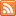# Content Tagged “Rounding (Probability)”## Basic Probability Rules

Published: Sep 19th, 2013

View Lecture Slides with Transcript – Basic Probability Rules Part 1 (12:51) Slides 1-11 Part 2 (12:26) Slides 12-20 This document is linked from Basic Probability Rules.## Basic Probability Rules

Published: Jul 28th, 2012

Introduction Rules of Probability Probability Rule One (For any event A, 0 ≤ P(A) ≤ 1) Probability Rule Two (The sum of the probabilities of all possible outcomes is 1) Probability Rule […]